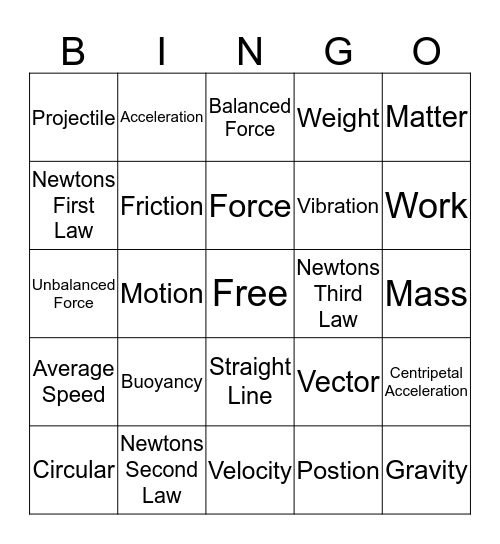# Force and Motion BINGOThis bingo card has a free space and 24 words: Buoyancy, Newtons Second Law, Force, Circular, Matter, Projectile, Newtons First Law, Acceleration, Newtons Third Law, Straight Line, Vibration, Unbalanced Force, Vector, Centripetal Acceleration, Friction, Postion, Mass, Balanced Force, Work, Motion, Gravity, Average Speed, Weight and Velocity.

## Play Online